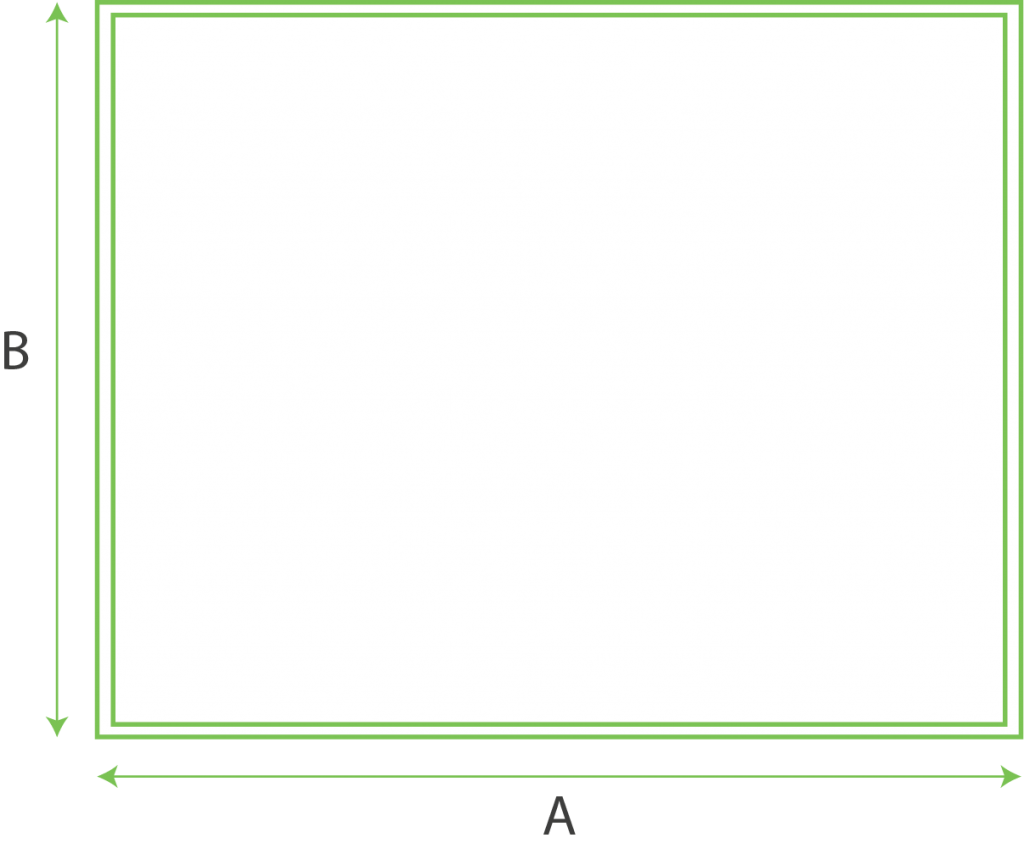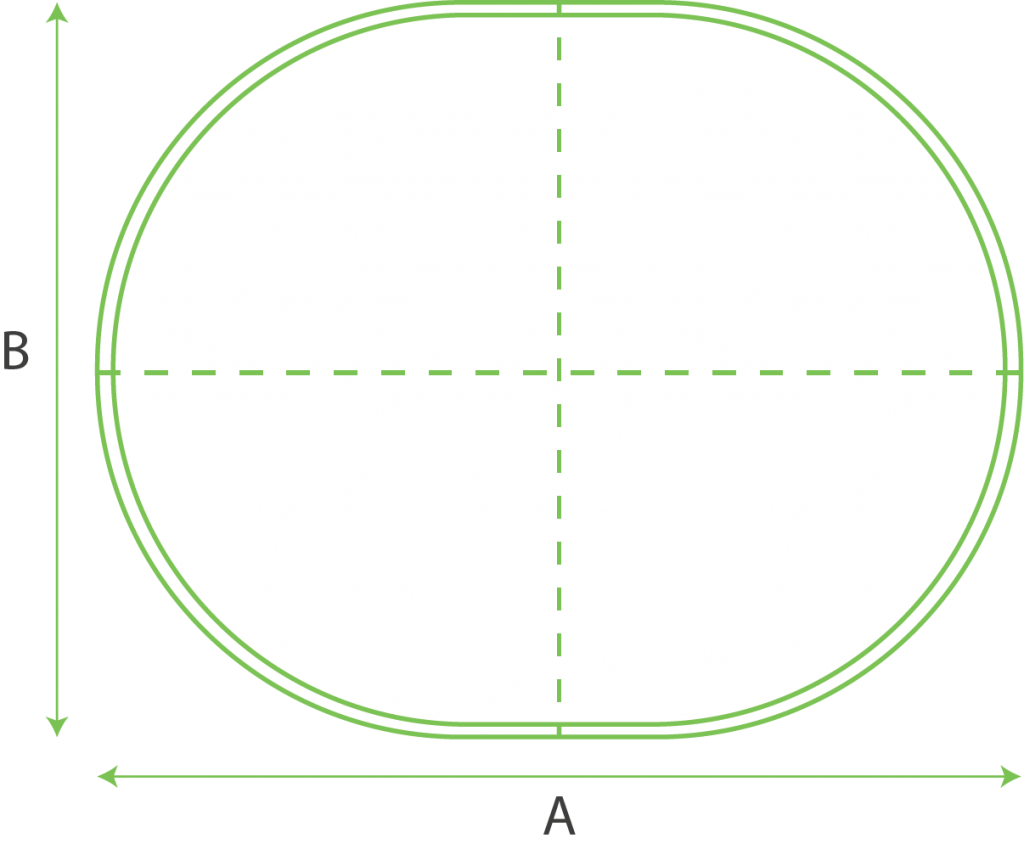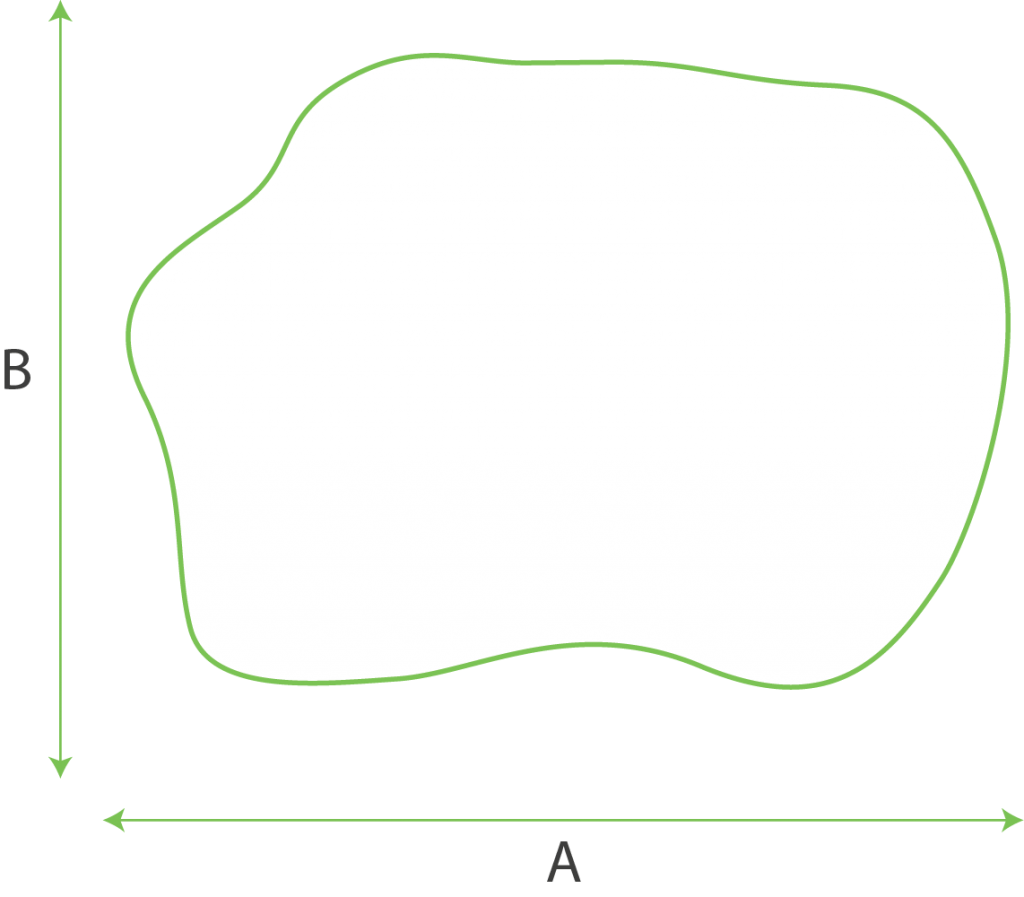# Measuring Guide## Square area

The amount of grass required for a square or rectangular area can be calculated by multiplying the width by the length.

## Calculation AxB## Round or oval shaped area

The amount of grass required for a round area can easily be measured by multiplying the longest width down the centre and longest height (in metres) along the centre leaving you with an area in square metres.

## Calculation AxB## L shaped area

The amount of grass required for an angled area should simply be taken in two calculations. Split the area into a rectangle and square shape (as detailed in the image) then simple multiply the width by the length for each section and add them together.

## Calculation AxB## Triangular area garden

With a right angled area of a triangle multiply A x B and divide by two.

## Calculation AxB## Angled or uneven garden areas

The amount of grass required for an angled or oblong area should simply be taken from the longest linear width and longest linear length along the grain.

## Calculation AxB## Unusual shaped garden area

If the area you are laying artificial grass on is an unusual or non-uniform shape you should measure the longest linear width and longest linear length as shown in the image.

## Request Free Quotation

Once you’ve worked out the area you would like to transform click the button below to submit your project.